Test: Basic & Electric Circuits- 1

# Test: Basic & Electric Circuits- 1

Test Description

## 10 Questions MCQ Test Topicwise Question Bank for Electrical Engineering | Test: Basic & Electric Circuits- 1

Test: Basic & Electric Circuits- 1 for Electrical Engineering (EE) 2023 is part of Topicwise Question Bank for Electrical Engineering preparation. The Test: Basic & Electric Circuits- 1 questions and answers have been prepared according to the Electrical Engineering (EE) exam syllabus.The Test: Basic & Electric Circuits- 1 MCQs are made for Electrical Engineering (EE) 2023 Exam. Find important definitions, questions, notes, meanings, examples, exercises, MCQs and online tests for Test: Basic & Electric Circuits- 1 below.
Solutions of Test: Basic & Electric Circuits- 1 questions in English are available as part of our Topicwise Question Bank for Electrical Engineering for Electrical Engineering (EE) & Test: Basic & Electric Circuits- 1 solutions in Hindi for Topicwise Question Bank for Electrical Engineering course. Download more important topics, notes, lectures and mock test series for Electrical Engineering (EE) Exam by signing up for free. Attempt Test: Basic & Electric Circuits- 1 | 10 questions in 30 minutes | Mock test for Electrical Engineering (EE) preparation | Free important questions MCQ to study Topicwise Question Bank for Electrical Engineering for Electrical Engineering (EE) Exam | Download free PDF with solutions
 1 Crore+ students have signed up on EduRev. Have you?
Test: Basic & Electric Circuits- 1 - Question 1

### If the power supplied by the 20 V voltage source shown in figure below is 60 W then, the power supplied by the unknown circuit element (x) will be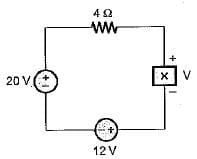Detailed Solution for Test: Basic & Electric Circuits- 1 - Question 1

we know that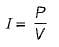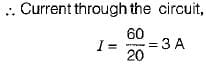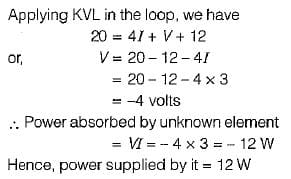Test: Basic & Electric Circuits- 1 - Question 2

### In the network of figure shown below, I1 = 0.05 A, I2 = 0.1 A , R = 50 Ω. The unknown current source has strength of i, If 100 J of energy is delivered to I2 in 1 min, the magnitude of i will be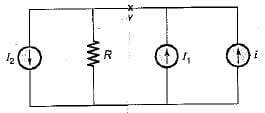Detailed Solution for Test: Basic & Electric Circuits- 1 - Question 2

energy dissipated in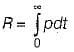where p=vi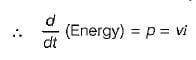Since power is constant, therefore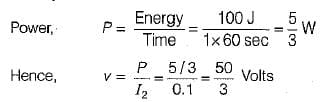Applying KCL at node v, we have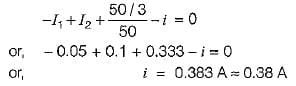Test: Basic & Electric Circuits- 1 - Question 3

### The energy required to move 120 C charge through 3 V is

Detailed Solution for Test: Basic & Electric Circuits- 1 - Question 3

W=QV=120 X 3 = 360J

Test: Basic & Electric Circuits- 1 - Question 4

For a given voltage, four heating coils will produce maximum heat, when connected

Detailed Solution for Test: Basic & Electric Circuits- 1 - Question 4

Maximum heat produced is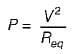for P to be maximum, Req should be minimum which is minimum of all four coils are connected in parallel.

Test: Basic & Electric Circuits- 1 - Question 5

Given figure shows a plot of d.c. voltage applied to a resistor of 5 Ω for 15 seconds. The total energy consumed by the resistor is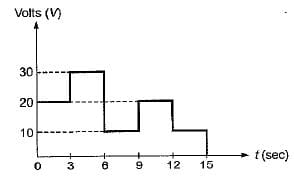Detailed Solution for Test: Basic & Electric Circuits- 1 - Question 5

energy = power x time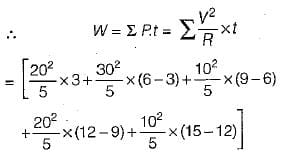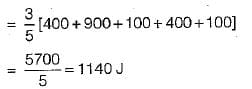Hence, the heat consumed by the resistor

= 1140 J

Test: Basic & Electric Circuits- 1 - Question 6

The charge in a capacitor is given by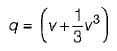if the voltage across this capacitor be v(t) = sin t, the current i(t) through the capacitor is

Detailed Solution for Test: Basic & Electric Circuits- 1 - Question 6

The current through.the capacitor is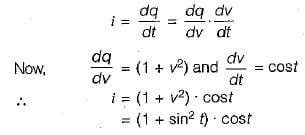Test: Basic & Electric Circuits- 1 - Question 7

The unit of reactive power is

Detailed Solution for Test: Basic & Electric Circuits- 1 - Question 7

Active power does do work, so it is the real axis. The unit for power is the watt (symbol: W). Apparent power is often expressed in volt-amperes (VA) since it is the product of rms voltage and rms current. The unit for reactive power is var, which stands for volt-ampere reactive.

Test: Basic & Electric Circuits- 1 - Question 8

The unit of electrical energy is

Test: Basic & Electric Circuits- 1 - Question 9

The given signal is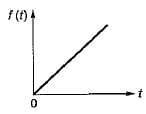Detailed Solution for Test: Basic & Electric Circuits- 1 - Question 9

Given signal is a ramp signal f(t) = A(t), which is neither an energy signal nor a power signal.

Test: Basic & Electric Circuits- 1 - Question 10

The two voltage sources connected in parallel as shown below, must satisfy the conditions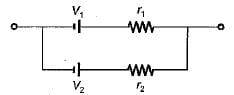## Topicwise Question Bank for Electrical Engineering

207 tests
Information about Test: Basic & Electric Circuits- 1 Page
In this test you can find the Exam questions for Test: Basic & Electric Circuits- 1 solved & explained in the simplest way possible. Besides giving Questions and answers for Test: Basic & Electric Circuits- 1, EduRev gives you an ample number of Online tests for practice

## Topicwise Question Bank for Electrical Engineering

207 tests(Scan QR code)